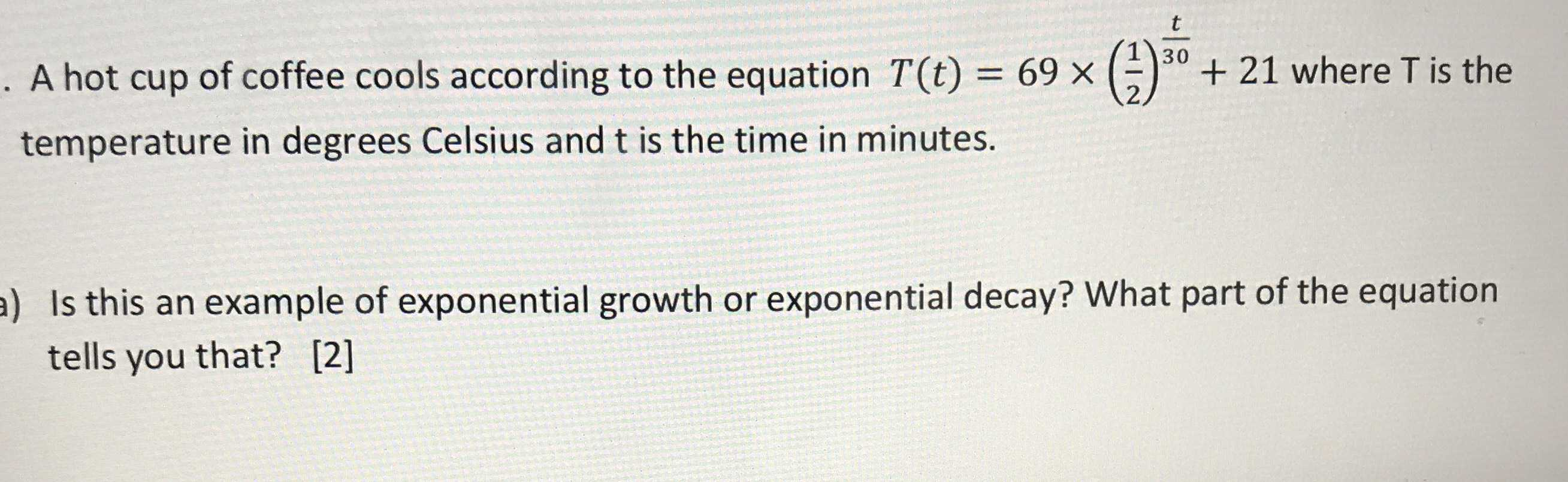### ¿Todavía tienes preguntas de matemáticas?

Pregunte a nuestros tutores expertos
Algebra
PreguntaA hot cup of coffee cools according to the equation $$T ( t ) = 69 \times ( \frac { 1 } { 2 } ) ^ { \frac { t } { 30 } } + 21$$ where $$T$$ is the temperature in degrees Celsius and $$t$$ is the time in minutes. Is this an example of exponential growth or exponential decay? What part of the equation tells you that?

The part of the equation that tells me this is $$\frac{1}{2}$$